# How to build a simple image/primary-colour similarity database

Writing proposals? Try Proppy, our new product!

We recently talked to some customers who are interested in finding images with similar colours in the context of fashion. There are several known solutions for this, and even some hosted services that do it for you.

Our case is a little bit different though because the images in the database change frequently. That rules out a fully supervised learning system due to the high cost of labelling new images all the time.

Anyway, here’s a small IPython notebook that explains the approach we chose. Note that this is only a very simple prototype that delivers a bad to OK experience. A fully tuned system takes advantage of training the ranker with click-stream data, uses a more human-friendly colour-space etc.

First we import all the libraries that we will need later:

In :
```%matplotlib inline
import matplotlib
import glob
import numpy
import pandas
from sklearn import cluster
from sklearn import decomposition
from sklearn import neighbors
from matplotlib import pyplot
from PIL import Image
```

To have some real data for testing we scraped images from the website http://oasis.andotherbrands.com/.

In :
```images = !find ./oasis -type f
images = sorted(images)
print "Number of images:", len(images)
images[:4]
```
```Number of images: 392
```
Out:
```['./oasis/3140031205.jpg',
'./oasis/3140031305.jpg',
'./oasis/3140031401.jpg',
'./oasis/3140031505.jpg']```

Let’s look at a random image:

In :
```im = [numpy.asarray(Image.open(x)) for x in images]
_ = pyplot.imshow(im)
```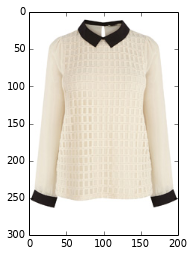There are several ways of extracting the primary colours in an image. Our constraints are:

• Unsupervised
• Can be applied to each image in isolation
• Fast

We went with simple k-means clustering.

We are going to flatten all images from a (n, m, 3) shape to a (n * m, 3) shape. I.e. instead of a two-dimensional space of (r, g, b) points we will have a one-dimensional space of (r, g, b) points. This is necessary because we care about the colours independently, in one big vector where order doesn’t matter. We do not care their relation to each other in the image.

In :
```flat_individual = [x.reshape(x.shape * x.shape, 3) for x in im if len(x.shape) == 3]
def primary_colours(x):
km = cluster.MiniBatchKMeans(5)
km.fit(x)
cc = km.cluster_centers_.copy()
return cc
features = [primary_colours(x) for x in flat_individual]
```

The result is 5 colours per image, e.g. for the image above:

In :
```im_0_colours = features.reshape(1, 5, 3) / 255
_ = pyplot.imshow(im_0_colours, interpolation='nearest')
```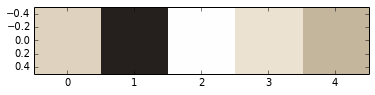To get an idea for more images we’ll paint the 5 primary colours for 20 images:

In :
```f, ax = pyplot.subplots(1)
f.set_size_inches(10, 10)
ax.set_title('5 primary colours per image')
_ = ax.imshow(numpy.array(features[20:40]).reshape(20, 5, 3) / 255, interpolation='nearest')
```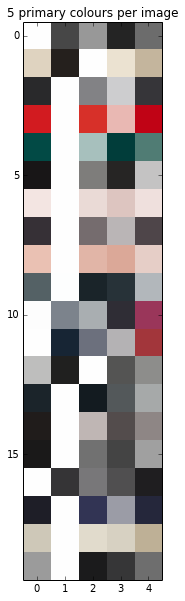One thing that sticks out immediately is that white shows up for every image. This is caused by the white background and will be important again when ranking.

Now that we have the five primary colours for every image we need a way of quickly looking up images by a given colour. Exact matches, e.g. `(255, 127, 99) == (255, 127, 99)`, are very unlikely because pixels in images are dependend on lighting, resolution etc. That means even when taking a picture of the same item twice it is unlikely to produce the exact same primary colours. One may be `(255, 127, 99)` and the other `(253, 129, 98)`.

The easiest way then is to do a nearest-neighbour search. These can be efficient, even in high dimensions using a library like FLANN but for our 3-dimensional colour lookup the standard scikit-learn version will do fine:

In :
```nd_features = numpy.array(features)
flat_features = nd_features.reshape(nd_features.shape * nd_features.shape, 3)
nns = neighbors.NearestNeighbors(5).fit(flat_features)
ds, ix = nns.kneighbors(flat_features[100:150], 20)
```

Training was fast, let’s graph the similarity of colours. We start with the lookup colour on the left side and paint the results from the nearest-neighbour search to the right, with decreasing similarity.

In :
```p = flat_features[ix].reshape(50, 20, 3)
f, ax = pyplot.subplots(1)
f.set_size_inches(10, 10)
ax.set_title('Colour similarty (less similar going to the right)')
_ = ax.imshow(p / p.max(), interpolation='nearest')
```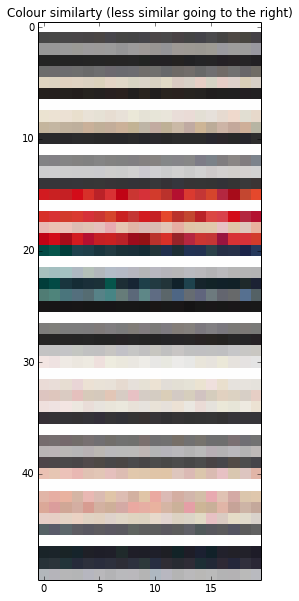That looks pretty sane.

Now we have:

1. A feature extractor (k-means clustering)
2. A feature index (nearest-neighbours)

The third and last step is to rank the results. Ranking is a complex subject in itself. We’re going to use a stupidly simple ranker to illustrate the point.

I want to highlight one little trick we are doing though: Remember that white was part of every picture because it is the background? This will reduce the search quality a lot because it makes images more similar than they should be according to their other colours.

In order to get around this white-is-everywhere problem we are borrowing a trick from text-indexing: tf-idf which stands for term-frequency, inverse-document-frequency. The basic idea can be summarized as “the more common a colour is among the images the less weight it should have”. I.e. white should have a low weight.

Note that we could simply have filtered out white but that solution is less general, and doesn’t work for fashion items with a light-blue background or unfortunate colour distributions.

In :
```# Cluster all colours to allow determining an inverse document frequency.
idf_kmeans = cluster.MiniBatchKMeans(60).fit(flat_features)
tf_idf_df = pandas.DataFrame()
tf_idf_df['idf_count'] = idf_kmeans.counts_[idf_kmeans.labels_]
tf_idf_df['idf'] = numpy.log(len(tf_idf_df) / tf_idf_df['idf_count'])
tf_idf_df['tf'] = 5
```

With the tf-idf information we can implement our simple ranker:

In :
```def rank(colours, tf_idf_df, nns):
# Find some candidates (10 per colour)
distances, ix = nns.kneighbors(colours, 10)
candidate_df = pandas.DataFrame(
dict(colour_distance=distances.flatten()),
index=ix.flatten(),
)
result = tf_idf_df.join(candidate_df)
# The following line decides the rank. Tuning and augmenting
# it with additional data can vastly improve results.
result['rank'] =  result['colour_distance'] / ( result['idf'] * result['tf'] )
return result.sort('rank').dropna()
```

We expect the first five colours to have exact matches (colour-distance 0), so we rank them but then cut them off:

In :
```ranked = rank(flat_features[5*21:5*21+5], tf_idf_df, nns)
```
Out:
idf_count idf tf colour_distance rank
1776 473 1.41905 5 0.021126 0.002977
1796 473 1.41905 5 0.055474 0.007818
1860 473 1.41905 5 0.068645 0.009675
131 473 1.41905 5 0.069630 0.009814
191 473 1.41905 5 0.080994 0.011415

Let’s plot some actual fashion items.

In :
```f, ax = pyplot.subplots(2, 5)
f.set_size_inches(10, 8)
for i, ax_ in enumerate(ax.ravel()):
ax_.imshow(im[ranked.irow(i + 4).name // 5]) # +4 because the first 5 items are perfect matchs.
```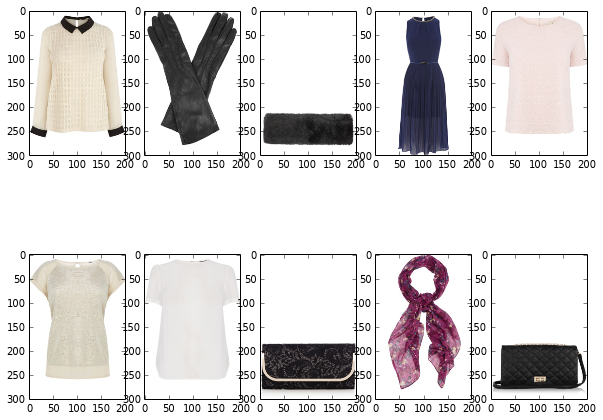We can see that this isn’t perfect but it’s already returning the cream-like and the darkish colours of the reference image (top left). The core algorithm now is:

1. Feature extraction
2. Indexing
3. Ranking

There is a lot of room for improvement in this simple pipeline. A few obvious choices are a better ranker or transforming to perception-friendly colour spaces like HSV. The three steps will stay the same though.